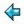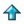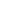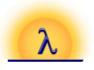### polish.m

Simple testbed for Miranda unification package, unify.m.

The expressions to be unified here are strings written in forward polish notation, such as "*+12-xy" - meaning (1+2)*(x-y). The operators are + - * / with single letter variables, and single digit constants. We provide bindings for the free identifiers of "unify.m" corresponding to this syntax.

```%include "unify.m"
{ expr==[char]; operator==char; var==char;
isvar=isvar; getvar=getvar; putvar=putvar;
rator=rator; rands=rands; construct=construct;
}

isvar e = letter (hd e)
getvar = hd
putvar = (:[])
rator = hd
rands (c:[]) = [], if digit c
rands (c:e) = [a,b],                  if member "+-*/" c & e2=[]
= error "illegal string", otherwise
where
(a,e1) = get e
(b,e2) = get e1
get [] = error "illegal string"
get (c:x) = ([c],x),        if letter c \/ digit c
= ([c]++a++b,x2), otherwise
where
(a,x1) = get x
(b,x2) = get x1
construct c xs = c:concat xs

test = unifyexprs "*+x3/7x" "*+1y/z1" ||the result should be "*+13/71"
test1 = unifyexprs "*+x3/7x" "*+1y/y1" ||not unifiable
```